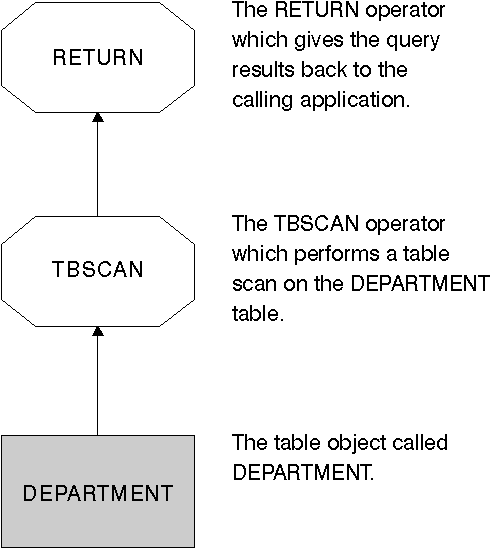## Introductory Concepts for Explain

You can use explain information to analyze the access plan that the optimizer has chosen based on the choices described in "Data Access Concepts and Optimization". For example, explain information may indicate that an index scan (see "Index Scan Concepts") was chosen by the optimizer. In addition, it can also allow you to determine the following:

As another example, the explain information could also help you understand how two tables are joined:

• The join method
• The order in which the tables are joined
• The occurrence and type of sorts.

Although you can use explain for SELECT, SELECT INTO, UPDATE, INSERT, VALUES, VALUES INTO, and DELETE SQL statements, the primary use of explain is to observe the access paths for the SELECT parts of your statements.

To satisfy an SQL query, the database manager typically:

• Uses one or more data objects (a table, an index, or both)
• Performs one or more operations (for example, table scan, index scan, and join)
• Returns the result set to the calling application.

For a simple SQL query, such as:

```   SELECT DEPTNO, DEPTNAME
FROM DEPARTMENT
```

the following, graphical representation of the steps performed could be displayed by Visual Explain:

Figure 51. Graphical Display of Explain OutputThe following topics discuss the type of details you can view for objects and operators:

### Explain Information for Data Objects

A single access plan may use one or more data objects to satisfy the SQL statement.

Object Statistics: The explain facility records facts about the object, such as:

• The creation time
• The last time that statistics were collected for the object (see Chapter 12. "System Catalog Statistics")
• An indication of whether or not the data in the object is ordered
• The number of columns in the object
• The estimated number of rows in the object
• The number of pages that the object occupies in the buffer pool
• The total estimated overhead, in milliseconds, for a single random I/O to the specified table space where this object is stored
• The estimated transfer rate, in milliseconds, to read a 4K page from the specified table space
• Prefetch and extent sizes, in 4K pages
• The degree of data clustering with the index
• The number of leaf pages used by this object's index and the number of levels in the tree
• The number of distinct full key values in this object's index
• The total number of overflow records in the table.

### Explain Information for Data Operators

A single access plan may perform several operations on the data to satisfy the SQL statement and provide results back to you. The SQL compiler determines the operations required; for example, a table scan, an index scan, a nested loop join, or a group-by. Details of many of these operators are provided in "Data Access Concepts and Optimization".

In addition to showing the various operators used in an access plan, explain information is also available for each operator as well as the cumulative effects of the access plan.

Estimated Cost Information: The following estimated, cumulative costs can be displayed for the operators. These costs are for the chosen access plan, up to and including the operator for which the information is captured.

• The total cost (in timerons)
• The number of 4KB page I/Os
• The number of CPU instructions
• The cost (in timerons) of fetching the first row, including any initial overhead required
• The communication cost (in frames).

Timerons are a made-up, relative unit of measure.

Operator Properties: The following information is recorded by the explain facility to describe the properties of each operator:

• The set of tables that have been accessed
• The set of columns that have been accessed
• The columns on which the data is ordered, if the optimizer determined that this ordering can be used by subsequent operators
• The set of predicates that have been applied
• The estimated number of rows that will be returned (cardinality).

[ Top of Page | Previous Page | Next Page | Table of Contents | Index ]

[ DB2 List of Books | Search the DB2 Books ]